作者：小傅哥 博客：https://bugstack.cn

## 一、前言

1. 数据插入流程和源码分析
2. 链表树化以及树转链表
3. 遍历过程中的无序Set的核心知识

🕵注意： 建议阅读上一篇后，再阅读本篇文章《HashMap核心知识，扰动函数、负载因子、扩容链表拆分，深度学习》

## 二、HashMap源码分析

### 1. 插入

#### 1.1 疑问点&考题

1. 如果出现哈希值计算的下标碰撞了怎么办？
2. 如果碰撞了是扩容数组还是把值存成链表结构，让一个节点有多个值存放呢？
3. 如果存放的数据的链表过长，就失去了散列表的性能了，怎么办呢？
4. 如果想解决链表过长，什么时候使用树结构呢，使用哪种树呢？

#### 1.2 插入流程和源码分析

HashMap插入数据流程图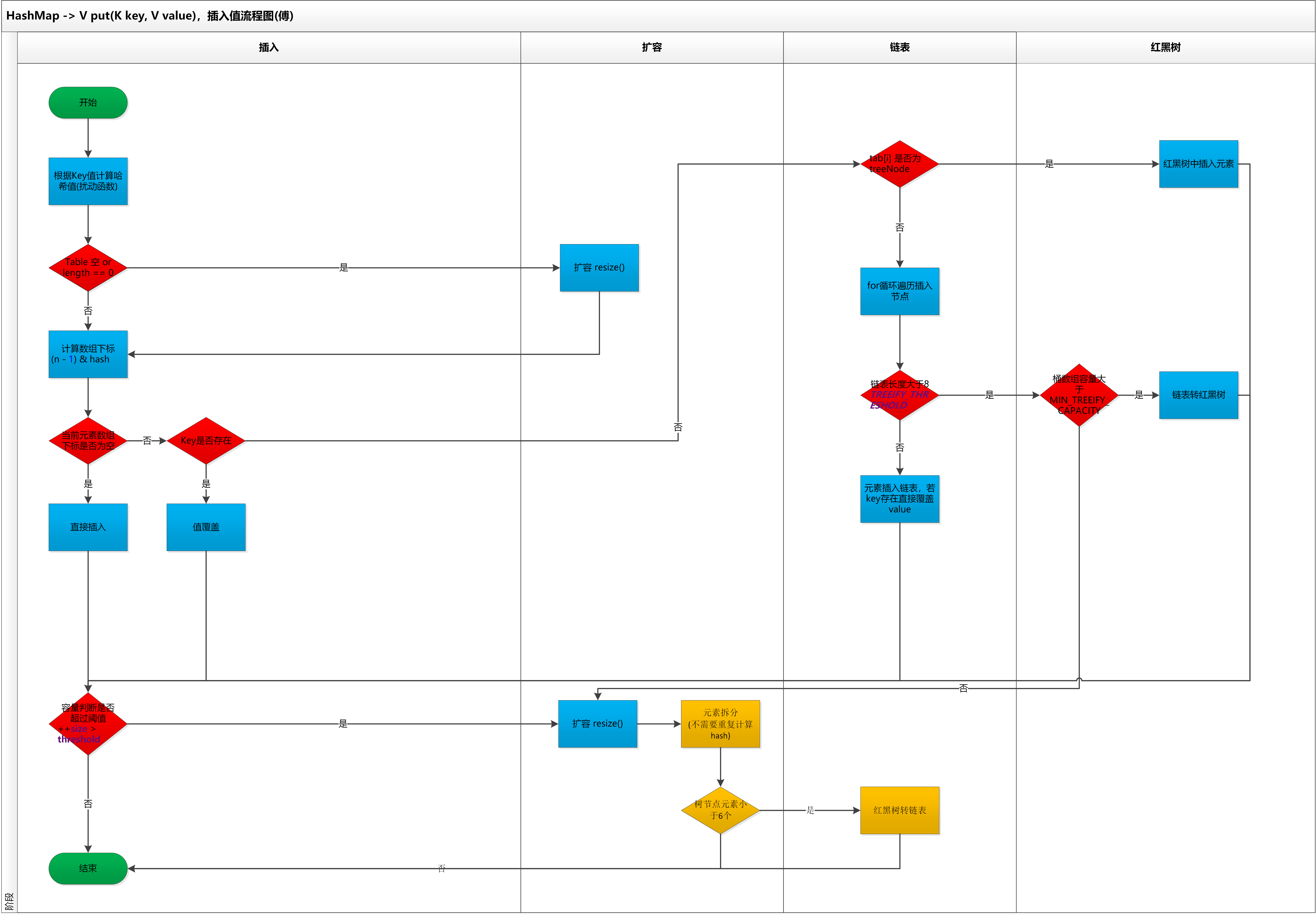visio原版流程图，可以通过关注公众号：bugstack虫洞栈，进行下载

1. 首先进行哈希值的扰动，获取一个新的哈希值。(key == null) ? 0 : (h = key.hashCode()) ^ (h >>> 16);

2. 判断tab是否位空或者长度为0，如果是则进行扩容操作。

if ((tab = table) == null || (n = tab.length) == 0)
n = (tab = resize()).length;
3. 根据哈希值计算下标，如果对应小标正好没有存放数据，则直接插入即可否则需要覆盖。tab[i = (n - 1) & hash])

4. 判断tab[i]是否为树节点，否则向链表中插入数据，是则向树中插入节点。

5. 如果链表中插入节点的时候，链表长度大于等于8，则需要把链表转换为红黑树。treeifyBin(tab, hash);

6. 最后所有元素处理完成后，判断是否超过阈值；threshold，超过则扩容。

7. treeifyBin,是一个链表转树的方法，但不是所有的链表长度为8后都会转成树，还需要判断存放key值的数组桶长度是否小于64 MIN_TREEIFY_CAPACITY。如果小于则需要扩容，扩容后链表上的数据会被拆分散列的相应的桶节点上，也就把链表长度缩短了。

JDK1.8 HashMap的put方法源码如下:

public V put(K key, V value) {
return putVal(hash(key), key, value, false, true);
}

final V putVal(int hash, K key, V value, boolean onlyIfAbsent,
boolean evict) {
Node<K,V>[] tab; Node<K,V> p; int n, i;
// 初始化桶数组 table，table 被延迟到插入新数据时再进行初始化
if ((tab = table) == null || (n = tab.length) == 0)
n = (tab = resize()).length;
// 如果桶中不包含键值对节点引用，则将新键值对节点的引用存入桶中即可
if ((p = tab[i = (n - 1) & hash]) == null)
tab[i] = newNode(hash, key, value, null);
else {
Node<K,V> e; K k;
// 如果键的值以及节点 hash 等于链表中的第一个键值对节点时，则将 e 指向该键值对
if (p.hash == hash &&
((k = p.key) == key || (key != null && key.equals(k))))
e = p;

// 如果桶中的引用类型为 TreeNode，则调用红黑树的插入方法
else if (p instanceof TreeNode)
e = ((TreeNode<K,V>)p).putTreeVal(this, tab, hash, key, value);
else {
// 对链表进行遍历，并统计链表长度
for (int binCount = 0; ; ++binCount) {
// 链表中不包含要插入的键值对节点时，则将该节点接在链表的最后
if ((e = p.next) == null) {
p.next = newNode(hash, key, value, null);
// 如果链表长度大于或等于树化阈值，则进行树化操作
if (binCount >= TREEIFY_THRESHOLD - 1) // -1 for 1st
treeifyBin(tab, hash);
break;
}

// 条件为 true，表示当前链表包含要插入的键值对，终止遍历
if (e.hash == hash &&
((k = e.key) == key || (key != null && key.equals(k))))
break;
p = e;
}
}

// 判断要插入的键值对是否存在 HashMap 中
if (e != null) { // existing mapping for key
V oldValue = e.value;
// onlyIfAbsent 表示是否仅在 oldValue 为 null 的情况下更新键值对的值
if (!onlyIfAbsent || oldValue == null)
e.value = value;
afterNodeAccess(e);
return oldValue;
}
}
++modCount;
// 键值对数量超过阈值时，则进行扩容
if (++size > threshold)
resize();
afterNodeInsertion(evict);
return null;
}

#### 1.3 扩容机制

HashMap是基于数组+链表和红黑树实现的，但用于存放key值得的数组桶的长度是固定的，由初始化决定。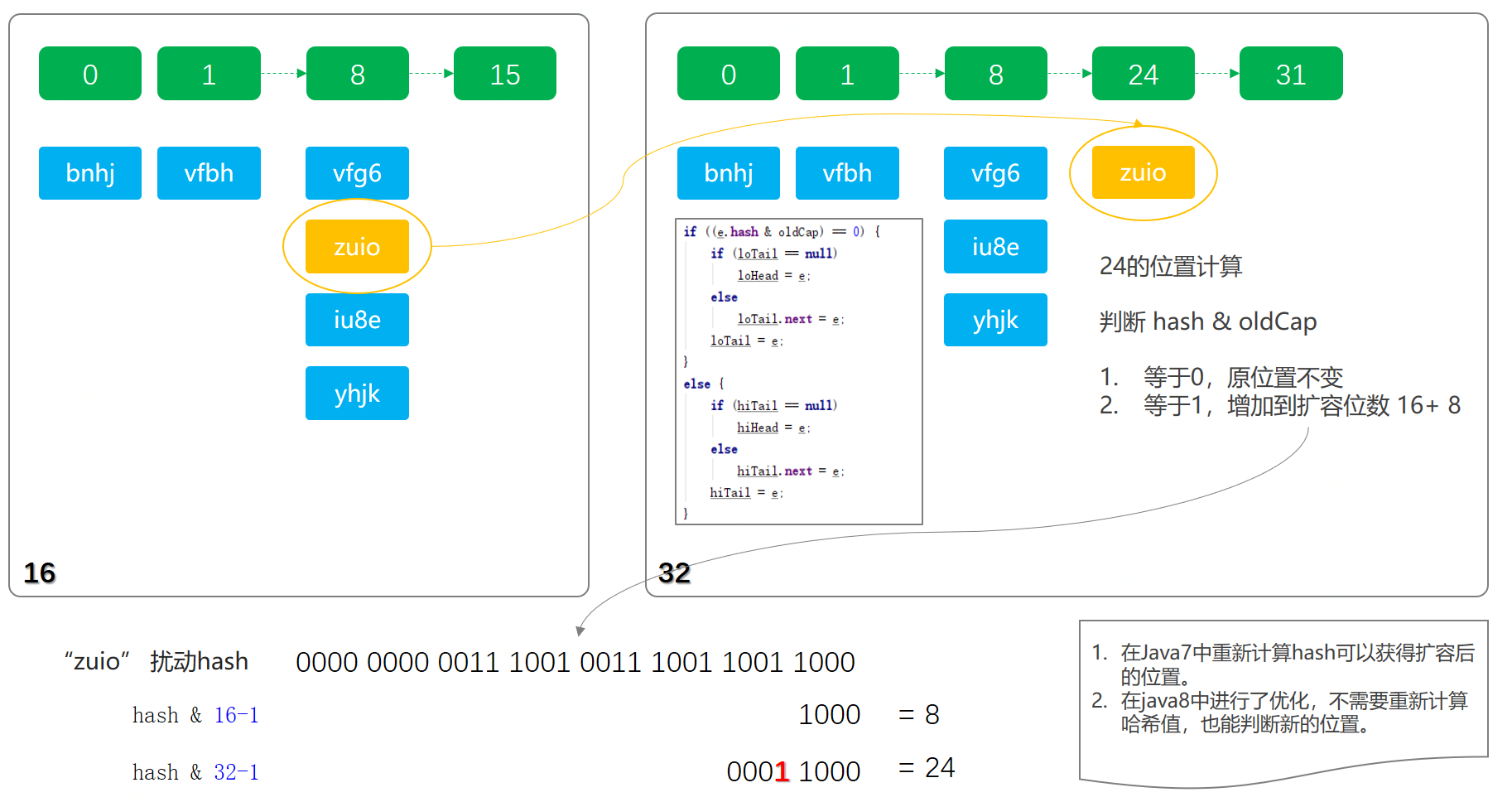final Node<K,V>[] resize() {
Node<K,V>[] oldTab = table;
int oldCap = (oldTab == null) ? 0 : oldTab.length;
int oldThr = threshold;
int newCap, newThr = 0;
// Cap 是 capacity 的缩写，容量。如果容量不为空，则说明已经初始化。
if (oldCap > 0) {
// 如果容量达到最大1 << 30则不再扩容
if (oldCap >= MAXIMUM_CAPACITY) {
threshold = Integer.MAX_VALUE;
return oldTab;
}

// 按旧容量和阀值的2倍计算新容量和阀值
else if ((newCap = oldCap << 1) < MAXIMUM_CAPACITY &&
oldCap >= DEFAULT_INITIAL_CAPACITY)
newThr = oldThr << 1; // double threshold
}
else if (oldThr > 0) // initial capacity was placed in threshold

// initial capacity was placed in threshold 翻译过来的意思，如下；
// 初始化时，将 threshold 的值赋值给 newCap，
// HashMap 使用 threshold 变量暂时保存 initialCapacity 参数的值
newCap = oldThr;
else {               // zero initial threshold signifies using defaults
// 这一部分也是，源代码中也有相应的英文注释
// 调用无参构造方法时，数组桶数组容量为默认容量 1 << 4; aka 16
// 阀值；是默认容量与负载因子的乘积，0.75
newCap = DEFAULT_INITIAL_CAPACITY;
}

// newThr为0，则使用阀值公式计算容量
if (newThr == 0) {
float ft = (float)newCap * loadFactor;
newThr = (newCap < MAXIMUM_CAPACITY && ft < (float)MAXIMUM_CAPACITY
(int)ft : Integer.MAX_VALUE);
}
threshold = newThr;

@SuppressWarnings({"rawtypes","unchecked"})
// 初始化数组桶，用于存放key
Node<K,V>[] newTab = (Node<K,V>[])new Node[newCap];
table = newTab;
if (oldTab != null) {
// 如果旧数组桶，oldCap有值，则遍历将键值映射到新数组桶中
for (int j = 0; j < oldCap; ++j) {
Node<K,V> e;
if ((e = oldTab[j]) != null) {
oldTab[j] = null;
if (e.next == null)
newTab[e.hash & (newCap - 1)] = e;
else if (e instanceof TreeNode)
// 这里split，是红黑树拆分操作。在重新映射时操作的。
((TreeNode<K,V>)e).split(this, newTab, j, oldCap);
else { // preserve order
Node<K,V> loHead = null, loTail = null;
Node<K,V> hiHead = null, hiTail = null;
Node<K,V> next;
// 这里是链表，如果当前是按照链表存放的，则将链表节点按原顺序进行分组{这里有专门的文章介绍，如何不需要重新计算哈希值进行拆分《HashMap核心知识，扰动函数、负载因子、扩容链表拆分，深度学习》}
do {
next = e.next;
if ((e.hash & oldCap) == 0) {
if (loTail == null)
else
loTail.next = e;
loTail = e;
}
else {
if (hiTail == null)
else
hiTail.next = e;
hiTail = e;
}
} while ((e = next) != null);

// 将分组后的链表映射到桶中
if (loTail != null) {
loTail.next = null;
}
if (hiTail != null) {
hiTail.next = null;
}
}
}
}
}
return newTab;
}

1. 扩容时计算出新的newCap、newThr，这是两个单词的缩写，一个是Capacity ，另一个是阀Threshold
2. newCap用于创新的数组桶 new Node[newCap];
3. 随着扩容后，原来那些因为哈希碰撞，存放成链表和红黑树的元素，都需要进行拆分存放到新的位置中。

#### 1.4 链表树化

HashMap这种散列表的数据结构，最大的性能在于可以O(1)时间复杂度定位到元素，但因为哈希碰撞不得已在一个下标里存放多组数据，那么jdk1.8之前的设计只是采用链表的方式进行存放，如果需要从链表中定位到数据时间复杂度就是O(n)，链表越长性能越差。因为在jdk1.8中把过长的链表也就是8个，优化为自平衡的红黑树结构，以此让定位元素的时间复杂度优化近似于O(logn)，这样来提升元素查找的效率。但也不是完全抛弃链表，因为在元素相对不多的情况下，链表的插入速度更快，所以综合考虑下设定阈值为8才进行红黑树转换操作。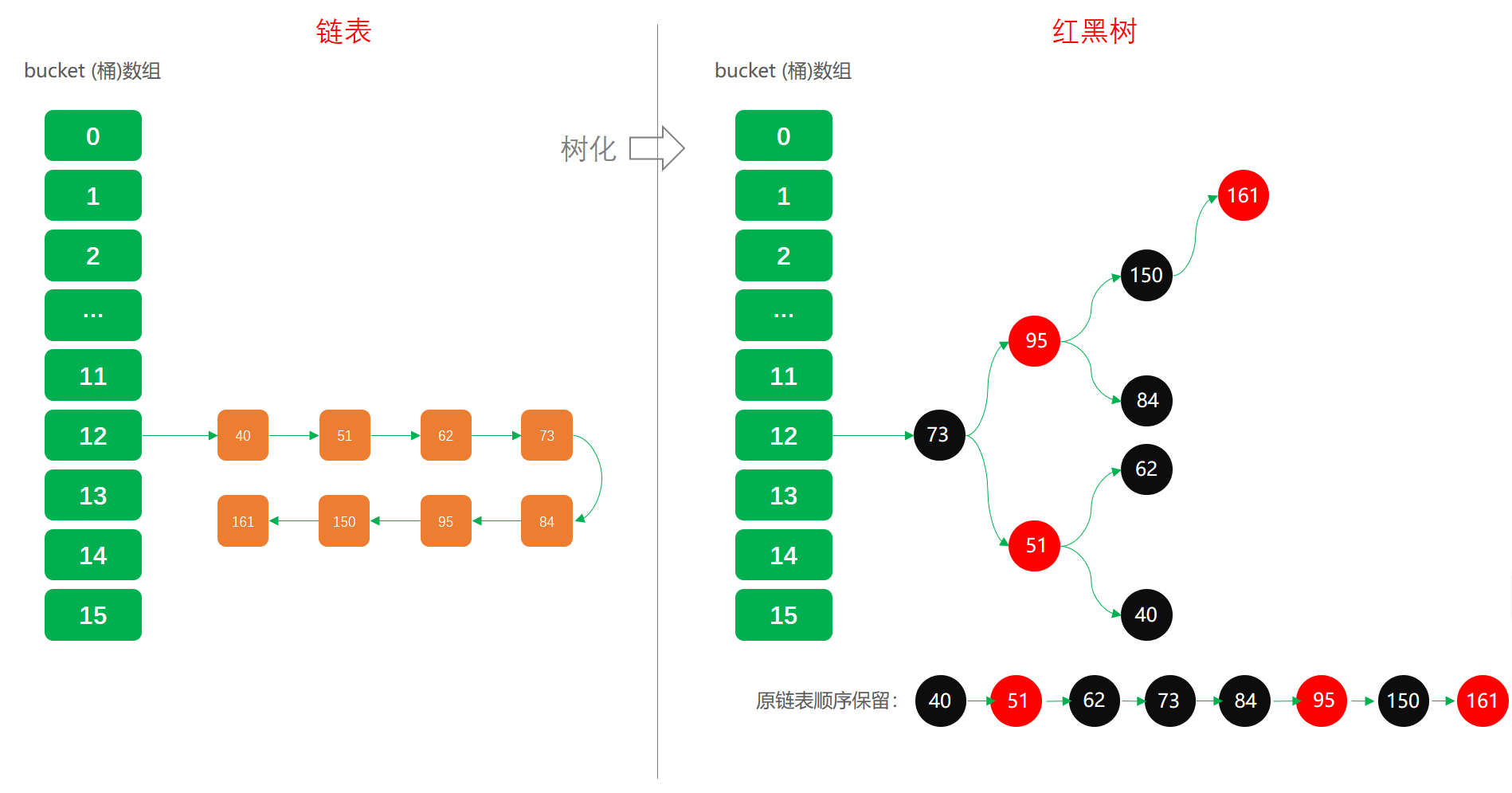final void treeifyBin(Node<K,V>[] tab, int hash) {
int n, index; Node<K,V> e;
// 这块就是我们上面提到的，不一定树化还可能只是扩容。主要桶数组容量是否小于64 MIN_TREEIFY_CAPACITY
if (tab == null || (n = tab.length) < MIN_TREEIFY_CAPACITY)
resize();
else if ((e = tab[index = (n - 1) & hash]) != null) {
// 又是单词缩写；hd = head (头部)，tl = tile (结尾)
TreeNode<K,V> hd = null, tl = null;
do {
// 将普通节点转换为树节点，但此时还不是红黑树，也就是说还不一定平衡
TreeNode<K,V> p = replacementTreeNode(e, null);
if (tl == null)
hd = p;
else {
p.prev = tl;
tl.next = p;
}
tl = p;
} while ((e = e.next) != null);
if ((tab[index] = hd) != null)
// 转红黑树操作，这里需要循环比较，染色、旋转。关于红黑树，在下一章节详细讲解
hd.treeify(tab);
}
}

1. 链表树化的条件有两点；链表长度大于等于8、桶容量大于64，否则只是扩容，不会树化。
2. 链表树化的过程中是先由链表转换为树节点，此时的树可能不是一颗平衡树。同时在树转换过程中会记录链表的顺序，tl.next = p，这主要方便后续树转链表和拆分更方便。
3. 链表转换成树完成后，在进行红黑树的转换。先简单介绍下，红黑树的转换需要染色和旋转，以及比对大小。在比较元素的大小中，有一个比较有意思的方法，tieBreakOrder加时赛，这主要是因为HashMap没有像TreeMap那样本身就有Comparator的实现。

#### 1.5 红黑树转链

final Node<K,V> untreeify(HashMap<K,V> map) {
Node<K,V> hd = null, tl = null;
// 遍历TreeNode
for (Node<K,V> q = this; q != null; q = q.next) {
// TreeNode替换Node
Node<K,V> p = map.replacementNode(q, null);
if (tl == null)
hd = p;
else
tl.next = p;
tl = p;
}
return hd;
}

// 替换方法
Node<K,V> replacementNode(Node<K,V> p, Node<K,V> next) {
return new Node<>(p.hash, p.key, p.value, next);
}

### 2. 查找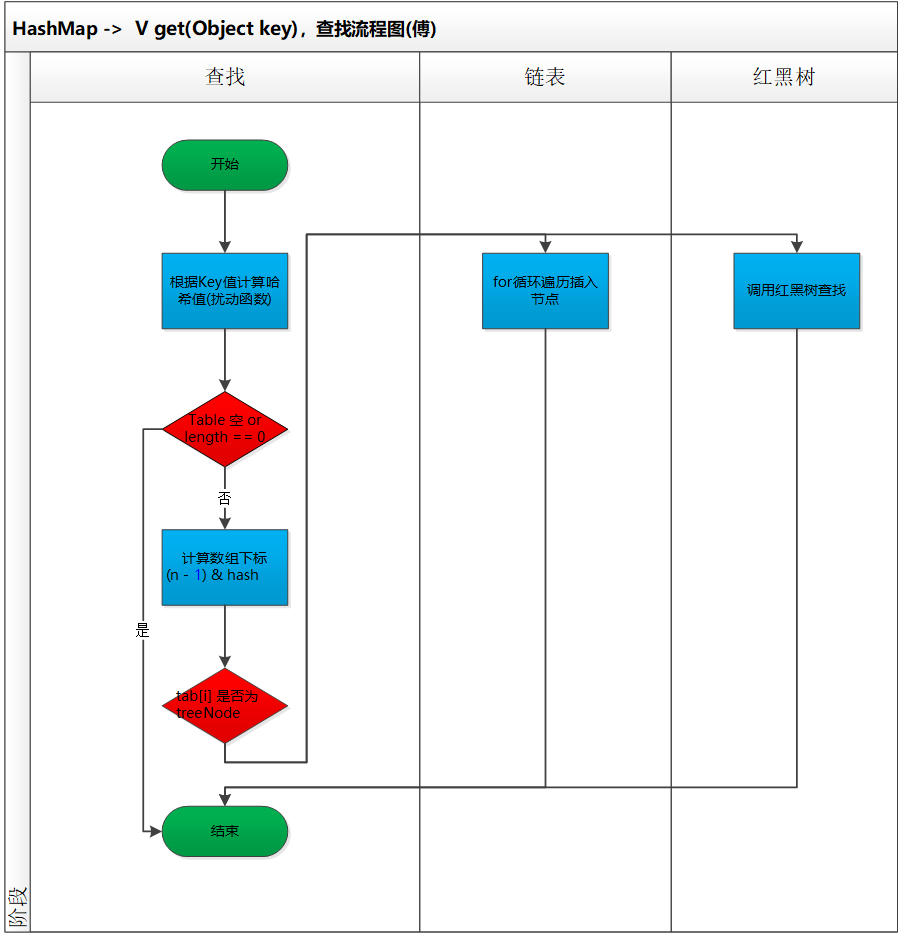public V get(Object key) {
Node<K,V> e;
// 同样需要经过扰动函数计算哈希值
return (e = getNode(hash(key), key)) == null ? null : e.value;
}

final Node<K,V> getNode(int hash, Object key) {
Node<K,V>[] tab; Node<K,V> first, e; int n; K k;
// 判断桶数组的是否为空和长度值
if ((tab = table) != null && (n = tab.length) > 0 &&
// 计算下标，哈希值与数组长度-1
(first = tab[(n - 1) & hash]) != null) {
if (first.hash == hash && // always check first node
((k = first.key) == key || (key != null && key.equals(k))))
return first;
if ((e = first.next) != null) {
// TreeNode 节点直接调用红黑树的查找方法，时间复杂度O(logn)
if (first instanceof TreeNode)
return ((TreeNode<K,V>)first).getTreeNode(hash, key);
// 如果是链表就依次遍历查找
do {
if (e.hash == hash &&
((k = e.key) == key || (key != null && key.equals(k))))
return e;
} while ((e = e.next) != null);
}
}
return null;
}

1. 扰动函数的使用，获取新的哈希值，这在上一章节已经讲过
2. 下标的计算，同样也介绍过 tab[(n - 1) & hash])
3. 确定了桶数组下标位置，接下来就是对红黑树和链表进行查找和遍历操作了

### 3. 删除

 public V remove(Object key) {
Node<K,V> e;
return (e = removeNode(hash(key), key, null, false, true)) == null ?
null : e.value;
}

final Node<K,V> removeNode(int hash, Object key, Object value,
boolean matchValue, boolean movable) {
Node<K,V>[] tab; Node<K,V> p; int n, index;
// 定位桶数组中的下标位置，index = (n - 1) & hash
if ((tab = table) != null && (n = tab.length) > 0 &&
(p = tab[index = (n - 1) & hash]) != null) {
Node<K,V> node = null, e; K k; V v;
// 如果键的值与链表第一个节点相等，则将 node 指向该节点
if (p.hash == hash &&
((k = p.key) == key || (key != null && key.equals(k))))
node = p;
else if ((e = p.next) != null) {
// 树节点，调用红黑树的查找方法，定位节点。
if (p instanceof TreeNode)
node = ((TreeNode<K,V>)p).getTreeNode(hash, key);
else {
// 遍历链表，找到待删除节点
do {
if (e.hash == hash &&
((k = e.key) == key ||
(key != null && key.equals(k)))) {
node = e;
break;
}
p = e;
} while ((e = e.next) != null);
}
}

// 删除节点，以及红黑树需要修复，因为删除后会破坏平衡性。链表的删除更加简单。
if (node != null && (!matchValue || (v = node.value) == value ||
(value != null && value.equals(v)))) {
if (node instanceof TreeNode)
((TreeNode<K,V>)node).removeTreeNode(this, tab, movable);
else if (node == p)
tab[index] = node.next;
else
p.next = node.next;
++modCount;
--size;
afterNodeRemoval(node);
return node;
}
}
return null;
} 
• 删除的操作也比较简单，这里面都没有太多的复杂的逻辑。
• 另外红黑树的操作因为被包装了，只看使用上也是很容易。

### 4. 遍历

#### 4.1 问题点

HashMap中的遍历也是非常常用的API方法，包括；

KeySet

 for (String key : map.keySet()) {
System.out.print(key + " ");
}

EntrySet

 for (HashMap.Entry entry : map.entrySet()) {
System.out.print(entry + " ");
}

#### 4.2 用代码测试

1. 这里我们要设定一个既有红黑树又有链表结构的数据场景
2. 为了可以有这样的数据结构，我们最好把HashMap的初始长度设定为64，避免在链表超过8位后扩容，而是直接让其转换为红黑树。
3. 找到18个元素，分别放在不同节点(这些数据通过程序计算得来)；
1. 桶数组02节点：24、46、68
2. 桶数组07节点：29
3. 桶数组12节点：150、172、194、271、293、370、392、491、590

@Test
public void test_Iterator() {
Map<String, String> map = new HashMap<String, String>(64);
map.put("24", "Idx：2");
map.put("46", "Idx：2");
map.put("68", "Idx：2");
map.put("29", "Idx：7");
map.put("150", "Idx：12");
map.put("172", "Idx：12");
map.put("194", "Idx：12");
map.put("271", "Idx：12");
System.out.println("排序01：");
for (String key : map.keySet()) {
System.out.print(key + " ");
}

map.put("293", "Idx：12");
map.put("370", "Idx：12");
map.put("392", "Idx：12");
map.put("491", "Idx：12");
map.put("590", "Idx：12");
System.out.println("\n\n排序02：");
for (String key : map.keySet()) {
System.out.print(key + " ");
}

map.remove("293");
map.remove("370");
map.remove("392");
map.remove("491");
map.remove("590");
System.out.println("\n\n排序03：");
for (String key : map.keySet()) {
System.out.print(key + " ");
}

}

1. 添加元素，在HashMap还是只链表结构时，输出测试结果01
2. 添加元素，在HashMap转换为红黑树时候，输出测试结果02
3. 删除元素，在HashMap转换为链表结构时，输出测试结果03

#### 4.3 测试结果分析

排序01：
24 46 68 29 150 172 194 271

24 46 68 29 271 150 172 194 293 370 392 491 590

24 46 68 29 172 271 150 194
Process finished with exit code 0

1. 01情况下，排序定位哈希值下标和链表信息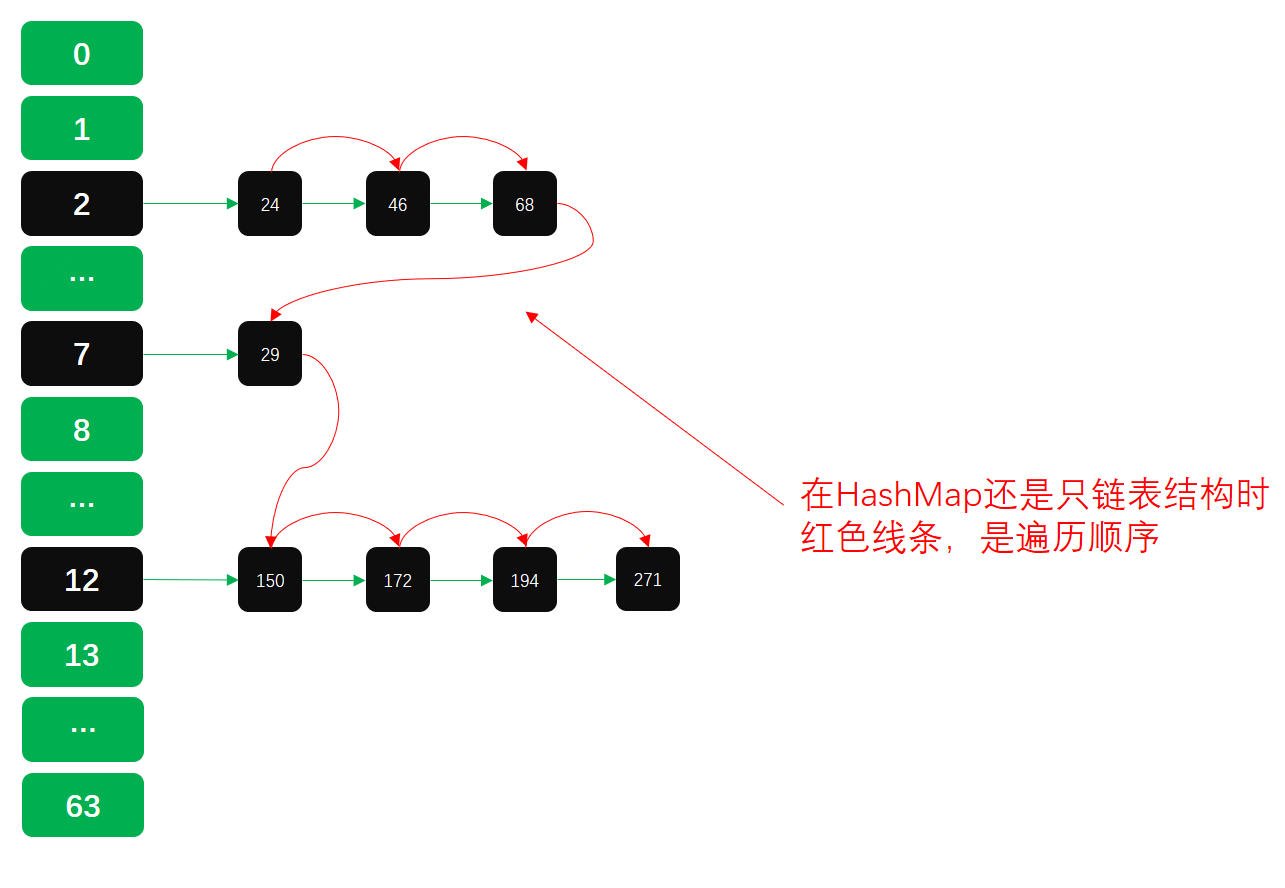1. 02情况下，因为链表转换为红黑树，树根会移动到数组头部。moveRootToFront()方法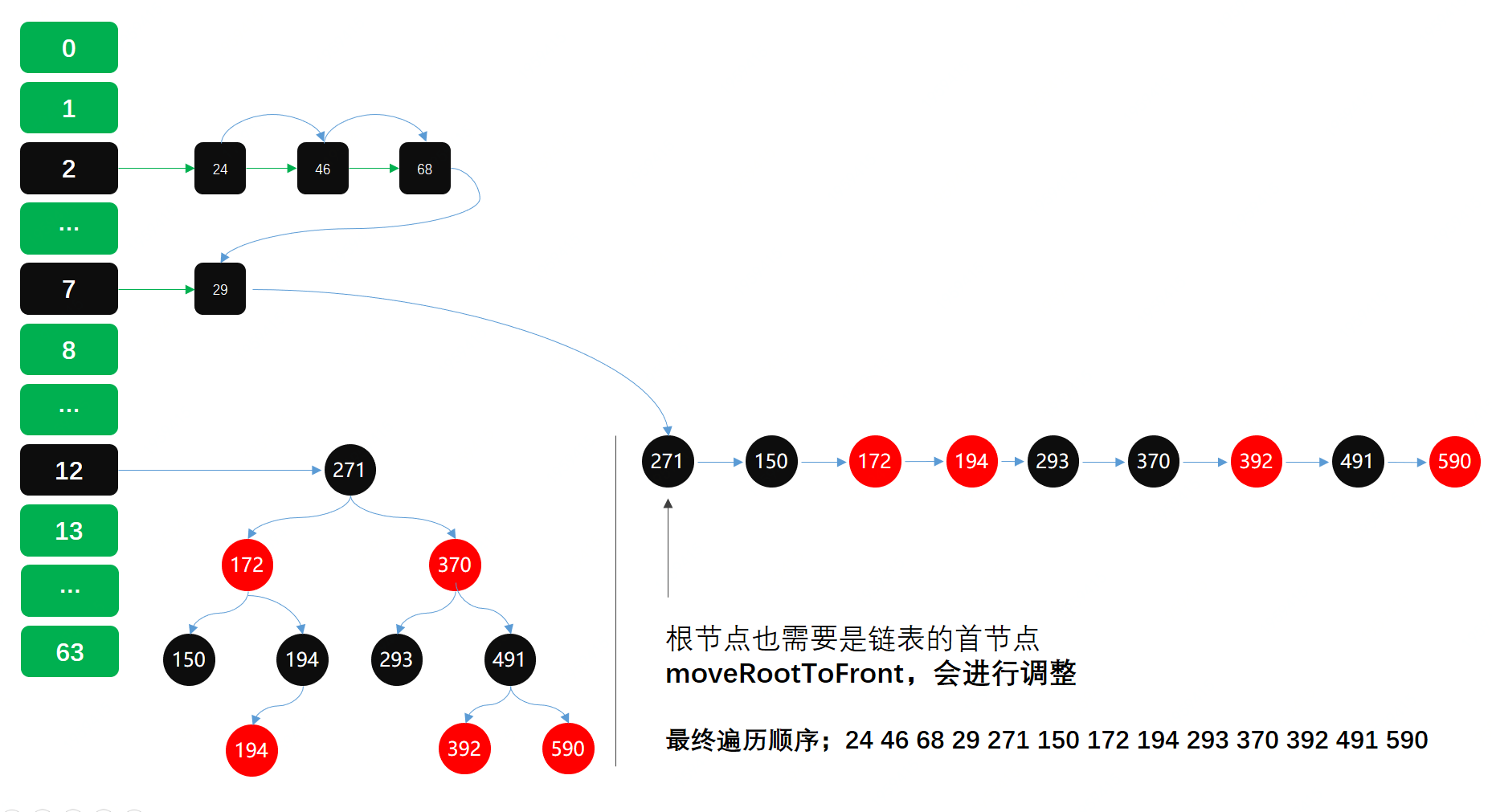1. 03情况下，因为删除了部分元素，红黑树退化成链表。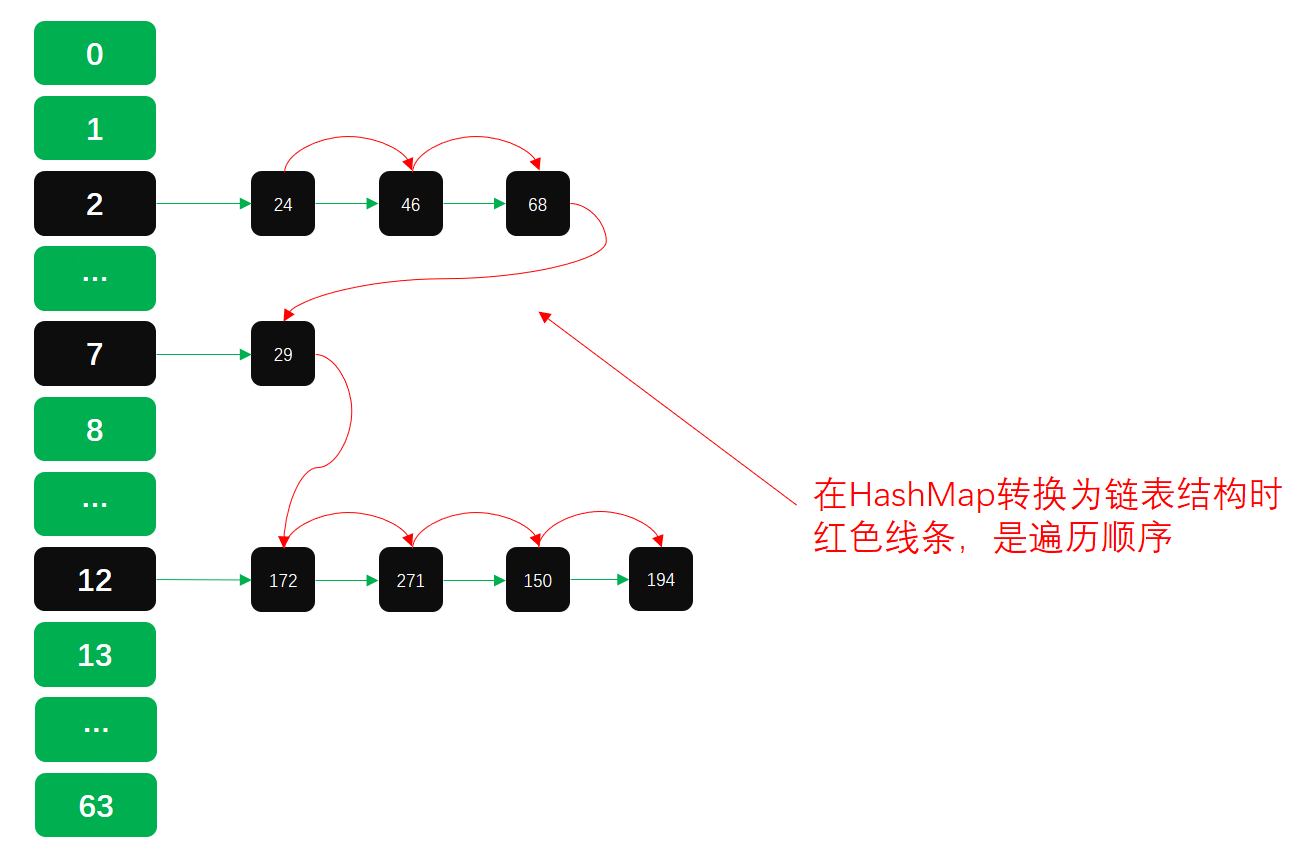## 三、总结

• 这一篇API源码以及逻辑与上一篇数据结构中扰动函数、负载因子、散列表实现等，内容的结合，算是把HashMap基本常用技术点，梳理完成了。但知识绝不止于此，这里还有红黑树的相关技术内容，后续会进行详细。
• 除了HashMap以外还有TreeMap、ConcurrentHashMap等，每一个核心类都有一些相关的核心知识点，每一个都非常值得深入研究。这个烧脑的过程，是学习获得知识的最佳方式。
• 可能关于HashMap还有一些疏漏的点，也希望阅读的小伙伴可以提出更多的问题，互相学习，共同进步，本文就到这里，感谢您的阅读！

## 四、推荐阅读